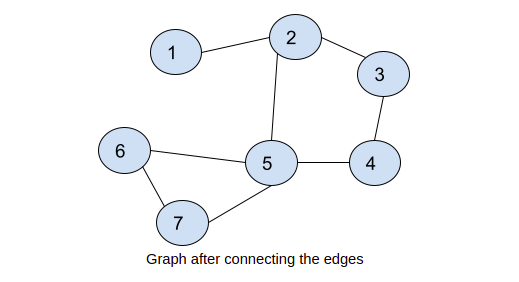# Count of Simple Cycles in a Connected Undirected Graph Having N Vertices

## Introduction

A cycle consists of at least three vertices connected in a loop through the edges. In an undirected graph, there can be multiple such cycles that may exist. Here, we will see how to count the number of such cycles present in the undirected graph.

## Problem statement

You are given a connected undirected graph; you need to count the number of simple cycles in the undirected graph.

Input:

edges= [(1, 2),(2, 3),(3, 4),(4, 5),(5, 2),(5, 6), (5, 7),(6, 7)]

Graph after connecting the edgesOutput:

number of cycles in the undirected graph: 2

Note: Please try to solve the problem first and then see the solution below.

## Approach

We will be using DFS and graph coloring properties to count the number of cycles in the undirected graph.

### Algorithm

• We will maintain a color[ ] array that stores the color of each vertex and par[ ] array that stores the parent of each vertex of the graph.
• We will use the DFS (depth-first-search) approach to visit every vertex of the graph.
• If any vertex is visited for the first time, it will be marked as partially visited along with its vertices connected with it.
• During a recursive DFS call for a vertex, if it is found partially visited, we will increment the count of the cycle number which stores the number of cycles in the undirected graph.
• If all connected nodes are visited from a vertex, then we will mark the vertex as fully visited.
• If any fully visited vertex is found, we will skip that node.

Output

## Complexity Analysis

Time Complexity

Here for finding the number of cycles in the undirected graph, time complexity is the same as DFS traversal, i.e., O(V+E), where V is the number of vertices and E is the number of edges in the graph.

Space complexity  is O(V) as extra color[ ] and par[ ]  is used here.

### What is a connected graph?

A graph is connected if there is a path between every pair of vertices in the graph.

### What is the difference between a directed and undirected graph?

The main difference between a directed and undirected graph is that edges in a directed graph have a specific direction (one-directional), but edges in an undirected graph are bidirectional.

### What are the approaches of graph traversal?

There is mainly two approaches are used to traverse a graph
DFS(depth-first-search)

### What is the application of graph coloring?

It is used in Sudoku problem solving, and map coloring for assigning mobile radio frequencies to towers.

## Conclusion

This article covered how to count the number of simple cycles in a connected undirected graph having N vertices.

Check out the CodeStudio library for getting a better hold of the data structures and algorithms.

Side by side, you can also practice a wide variety of coding questions commonly asked in interviews in CodeStudio. Along with coding questions, you can also find the interview experience of scholars working in renowned product-based companies here.

Happy learning!